# 5-7: Future Value of an Ordinary Annuity 5-8: Future Value of an Annuity Due

Future value of an annuity

Find the future values of these ordinary annuities. Compounding occurs once a year. Round your answers to the nearest cent.

1. \$200 per year for 4 years at 6%.

\$

2. \$100 per year for 2 years at 3%.

\$

3. \$1,000 per year for 16 years at 0%.

\$

4. Rework previous parts assuming that they are annuities due. Round your answers to the nearest cent.

5. \$200 per year for 4 years at 6%.

\$

6. \$100 per year for 2 years at 3%.

\$

7. \$1,000 per year for 16 years at 0%.

\$

This Homework Help Question: "5-7: Future Value of an Ordinary Annuity 5-8: Future Value of an Annuity Due" No answers yet.

We need 10 more requests to produce the answer to this homework help question. Share with your friends to get the answer faster!

0 /10 have requested the answer to this homework help question.

Once 10 people have made a request, the answer to this question will be available in 1-2 days.
All students who have requested the answer will be notified once they are available.

#### Earn Coin

Coins can be redeemed for fabulous gifts.

Similar Homework Help Questions
• ### FUTURE VALUE OF AN ANNUITY Find the future values of these ordinary annuities. Compounding occurs once...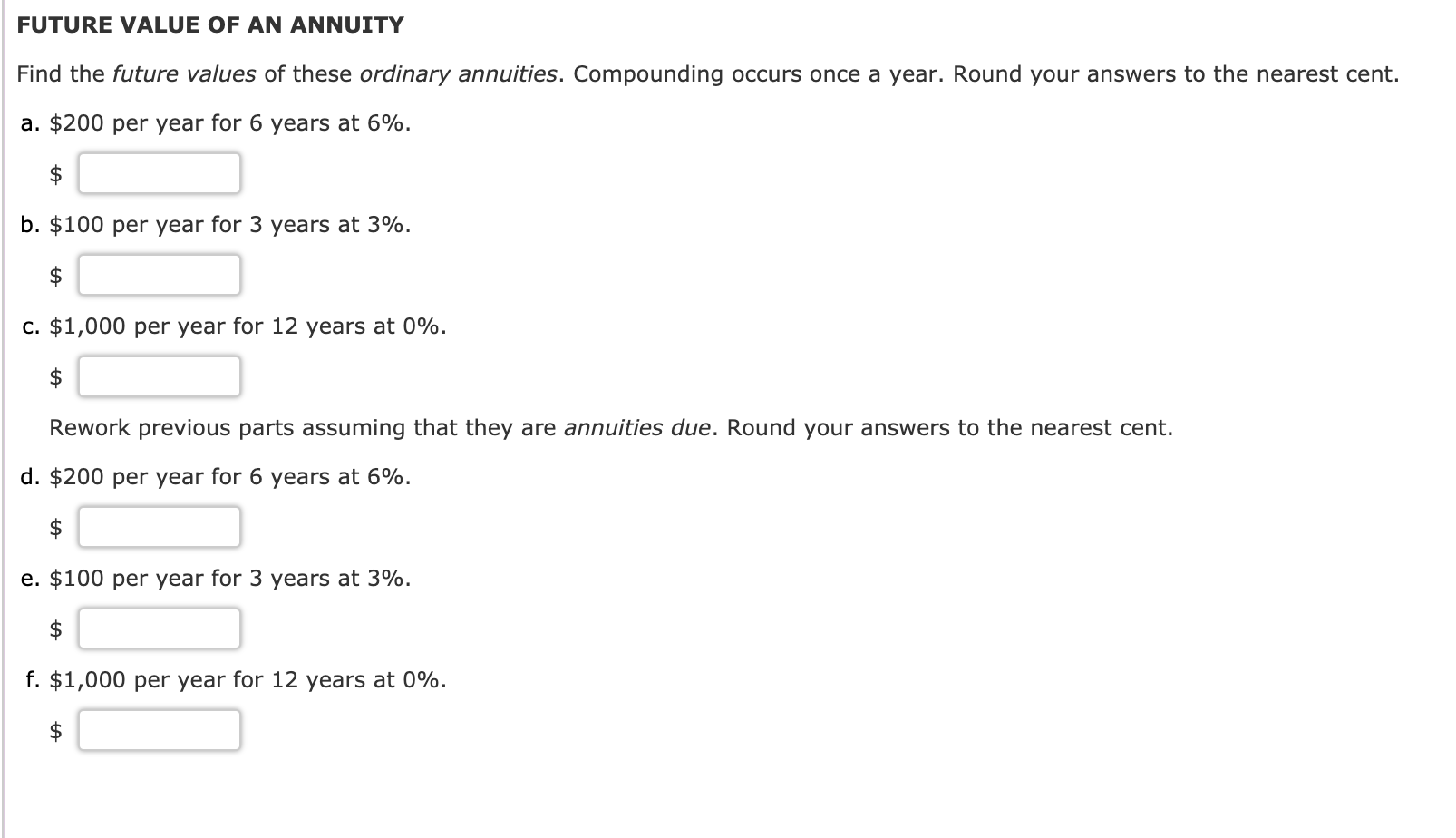FUTURE VALUE OF AN ANNUITY Find the future values of these ordinary annuities. Compounding occurs once a year. Round your answers to the nearest cent. a. \$200 per year for 6 years at 6%. \$ b. \$100 per year for 3 years at 3%. C. \$1,000 per year for 12 years at 0%. सी Rework previous parts assuming that they are annuities due. Round your answers to the nearest cent. d. \$200 per year for 6 years at 6%. e....

• ### FUTURE VALUE OF AN ANNUITY Find the future values of these ordinary annuities. Compounding occurs once...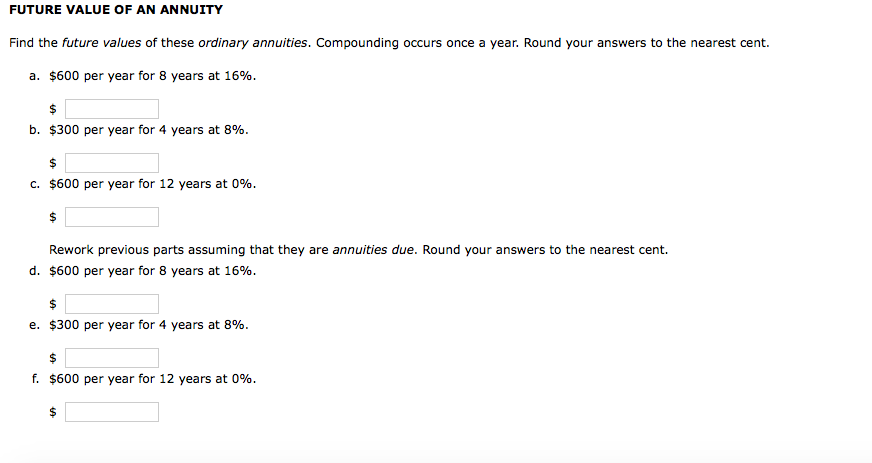FUTURE VALUE OF AN ANNUITY Find the future values of these ordinary annuities. Compounding occurs once a year. Round your answers to the nearest cent. a. \$600 per year for 8 years at 16%. b. \$300 per year for 4 years at 8%. c. \$600 per year for 12 years at 0%. Rework previous parts assuming that they are annuities due. Round your answers to the nearest cent. d, \$600 per year for 8 years at 16%. e. \$300 per...

• ### Find the future values of these ordinary annuities. Compounding occurs once a year. Round your an...

Find the future values of these ordinary annuities. Compounding occurs once a year. Round your answers to the nearest cent. 600 per year for 10 years at 16%. \$300 per year for 5 years at 8%. \$1,000 per year for 6 years at 0%. Rework previous parts assuming that they are annuities due. Round your answers to the nearest cent. \$600 per year for 10 years at 16%. \$300 per year for 5 years at 8%. \$1,000 per year for...

• ### Find the future values of these ordinary annuities. Compounding occurs once a year. Round your answers...

Find the future values of these ordinary annuities. Compounding occurs once a year. Round your answers to the nearest cent. \$800 per year for 2 years at 16%. \$400 per year for 1 year at 8%. \$1,000 per year for 16 years at 0%. Rework previous parts assuming that they are annuities due. Round your answers to the nearest cent. \$800 per year for 2 years at 16%. \$400 per year for 1 year at 8%. \$1,000 per year for...

• ### Find the future values of these ordinary annuities. Compounding occurs once a year. Do not round intermediate calculatio...

Find the future values of these ordinary annuities. Compounding occurs once a year. Do not round intermediate calculations. Round your answers to the nearest cent. \$400 per year for 14 years at 4%. \$200 per year for 7 years at 2%. \$1,000 per year for 7 years at 0%. Rework parts a, b, and c assuming they are annuities due. Future value of \$400 per year for 14 years at 4%: Future value of \$200 per year for 7 years...

• ### 5-14 FUTURE VALUE OF AN ANNUITY Find the future values of these ordinary annuities. Compounding occurs...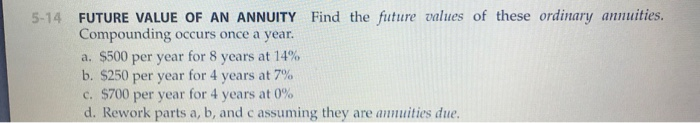5-14 FUTURE VALUE OF AN ANNUITY Find the future values of these ordinary annuities. Compounding occurs once a year. a. \$500 per year for 8 years at 14% b. \$250 per year for 4 years at 7% c. \$700 per year for 4 years at 0% d. Rework parts a, b, and c assuming they are annuities due.

• ### 8. Problem 5.15 (Present Value of an Annuity) eBook Find the present values of these ordinary...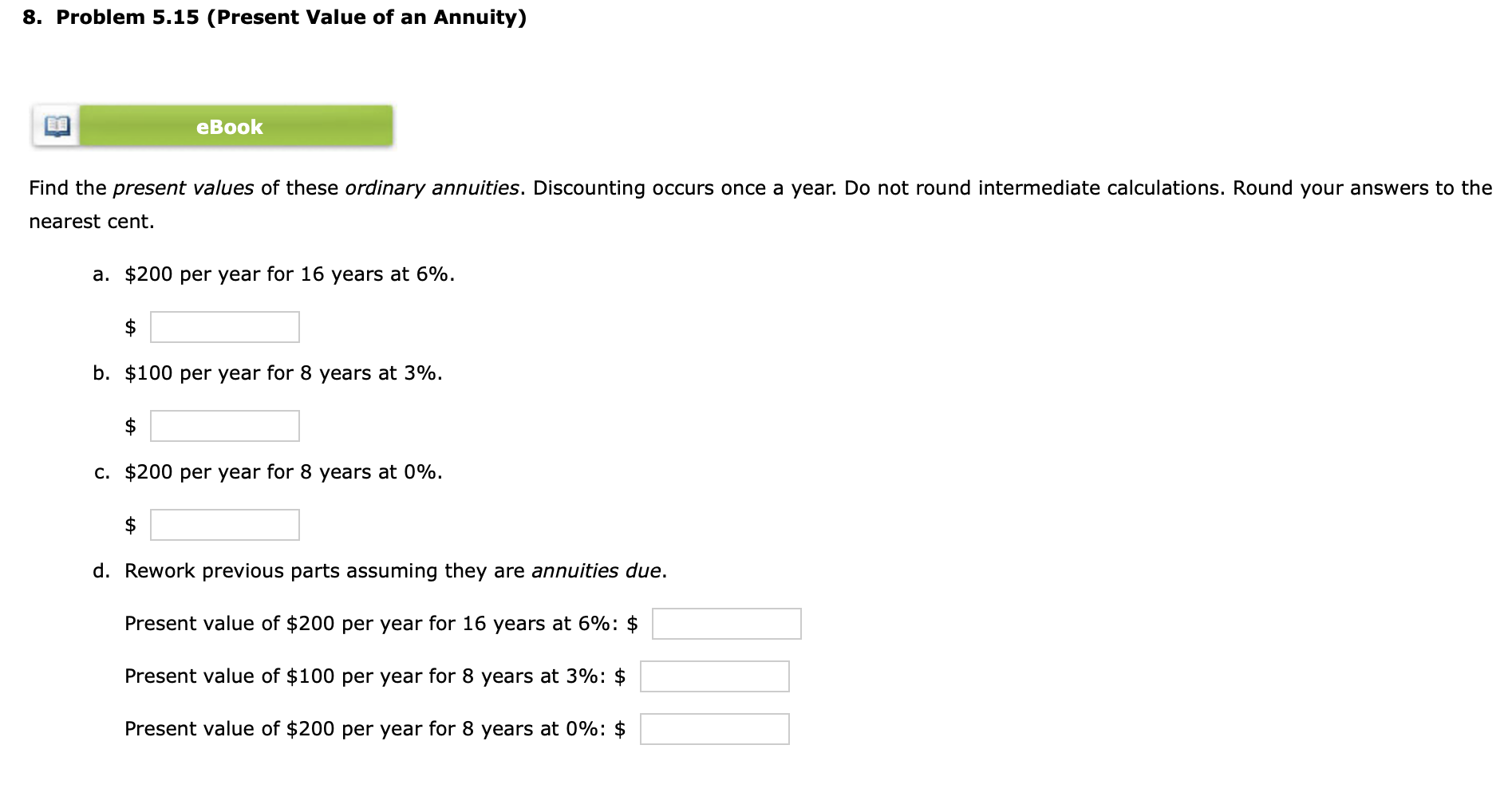8. Problem 5.15 (Present Value of an Annuity) eBook Find the present values of these ordinary annuities. Discounting occurs once a year. Do not round intermediate calculations. Round your answers to the nearest cent. a. \$200 per year for 16 years at 6%. b. \$100 per year for 8 years at 3%. C. \$200 per year for 8 years at 0%. d. Rework previous parts assuming they are annuities due. Present value of \$200 per year for 16 years at...

• ### 15. Problem 5.15 (Present Value of an Annuity) eBook Find the present values of these ordinary...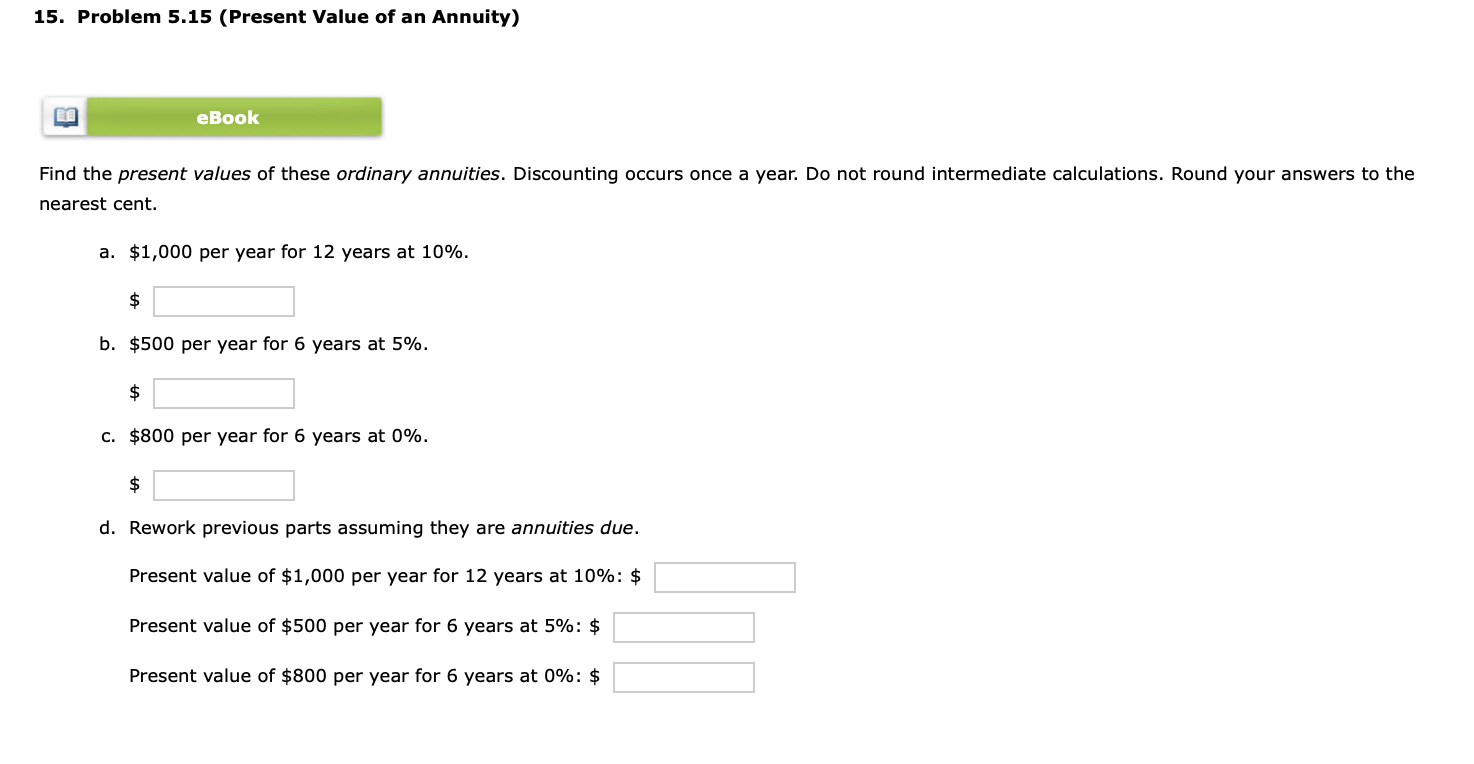15. Problem 5.15 (Present Value of an Annuity) eBook Find the present values of these ordinary annuities. Discounting occurs once a year. Do not round intermediate calculations. Round your answers to the nearest cent. a. \$1,000 per year for 12 years at 10%. b. \$500 per year for 6 years at 5%. c. \$800 per year for 6 years at 0%. d. Rework previous parts assuming they are annuities due. Present value of \$1,000 per year for 12 years at...

• ### Find the future values of these ordinary annuities. Compounding occurs once a year. Do not round...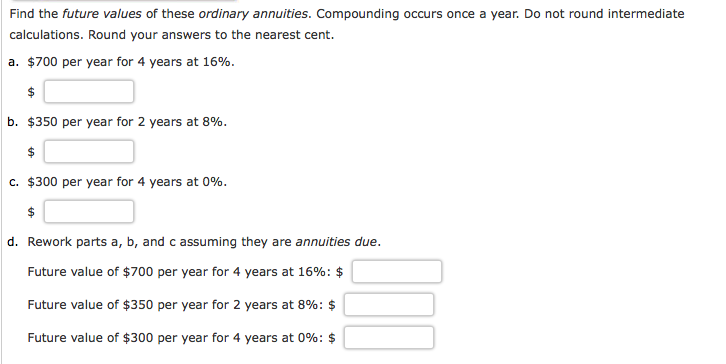Find the future values of these ordinary annuities. Compounding occurs once a year. Do not round intermediate calculations. Round your answers to the nearest cent. a. \$700 per year for 4 years at 16%. b. \$350 per year for 2 years at 8%. C. \$300 per year for 4 years at 096. d. Rework parts a, b, and c assuming they are annuities due. Future value of \$700 per year for 4 years at 16%: \$ Future value of \$350...

• ### eBook Find the future values of these ordinary annuities. Compounding occurs once a year. Do not...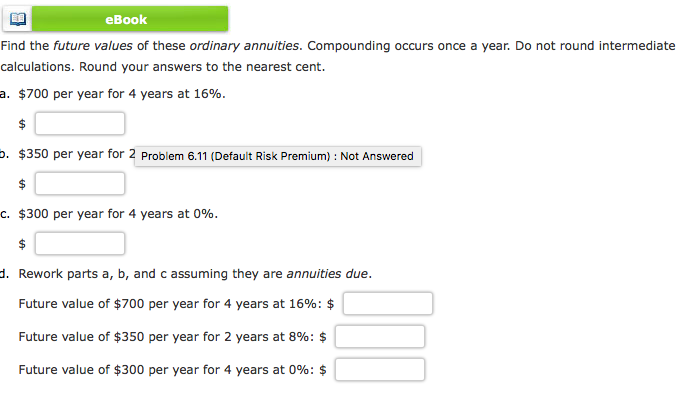eBook Find the future values of these ordinary annuities. Compounding occurs once a year. Do not round intermediate calculations. Round your answers to the nearest cent. a. \$700 per year for 4 years at 16%. \$350 per year for 2 Problem 6.11 (Default Risk Premium): Not Answered C. \$300 per year for 4 years at 0%. d. Rework parts a, b, and c assuming they are annuities due. Future value of \$700 per year for 4 years at 16% Future...